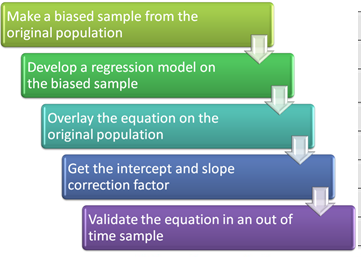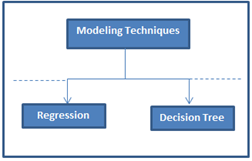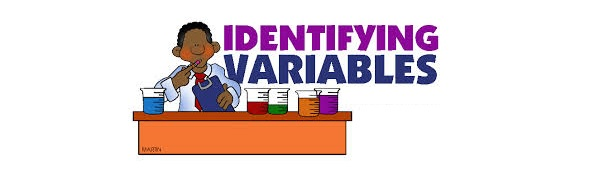## Comparing a CART model to Random Forest (Part 1)

I created my first simple regression model with my father in 8th standard (year: 2002) on MS Excel. Obviously, my contribution in that model was minimal, but I really enjoyed the graphical representation of the data. We tried validating all the assumptions etc. for this model. By the end of the exercise, we had 5 sheets of the simple regression model on 700 data points. The entire exercise was complex enough to confuse any person with average IQ level. When I look at my models today, which are## Framework to build logistic regression model in a rare event population

Only 531 out of a population of 50,431 customer closed their saving account in a year, but the dollar value lost because of such closures was more than \$ 5 Million.The best way to arrest these attrition was by predicting the propensity of attrition for individual customer and then pitch retention offers to these identified customers. This was a typical case of modeling in a rare event population. This kind of problems are also very common in Health care analytics.In such analysis, there are two## Trick to enhance power of Regression model

We, as analysts, specialize in optimization of already optimized processes. As the optimization gets finer, opportunity to make the process better gets thinner. One of the predictive modeling technique used frequently use is regression (Linear or Logistic). Another equally competing technique (typically considered as a challenger) is Decision tree.What if we could combine the benefits of both the techniques to create powerful predictive models?The trick mentioned in this article does exactly## Extracting right variables for your Regression model

Getting the right variables in your model and cleaning them can make or break your model.The precision of the model depends on the breadth (diversity) and depth (spread of data and correct transformations) of variables. This article will take you through some of the techniques used in the industry to create or transform variables. We will also cover the techniques used in the industry to select the right set of variables out of an exhaustive list created in our next article on the subject.TypesAssumptions of Linear Regression Model :There are number of assumptions of a linear regression model. In modeling, we normally check for five of the assumptions. These are as follows :1. Relationship between the outcomes and the predictors is linear. 2. Error term has mean almost equal to zero for each value of outcome. 3. Error term has constant variance. 4. Errors are uncorrelated. 5. Errors are normally distributed or we have an adequate sample size to rely on large sample theory.The point to